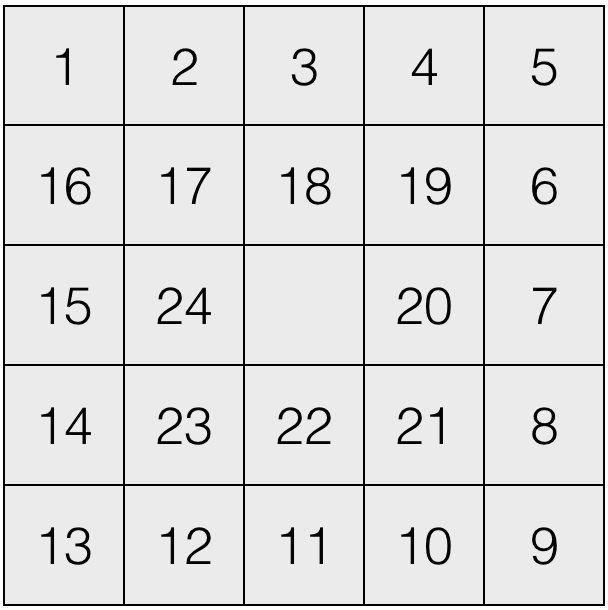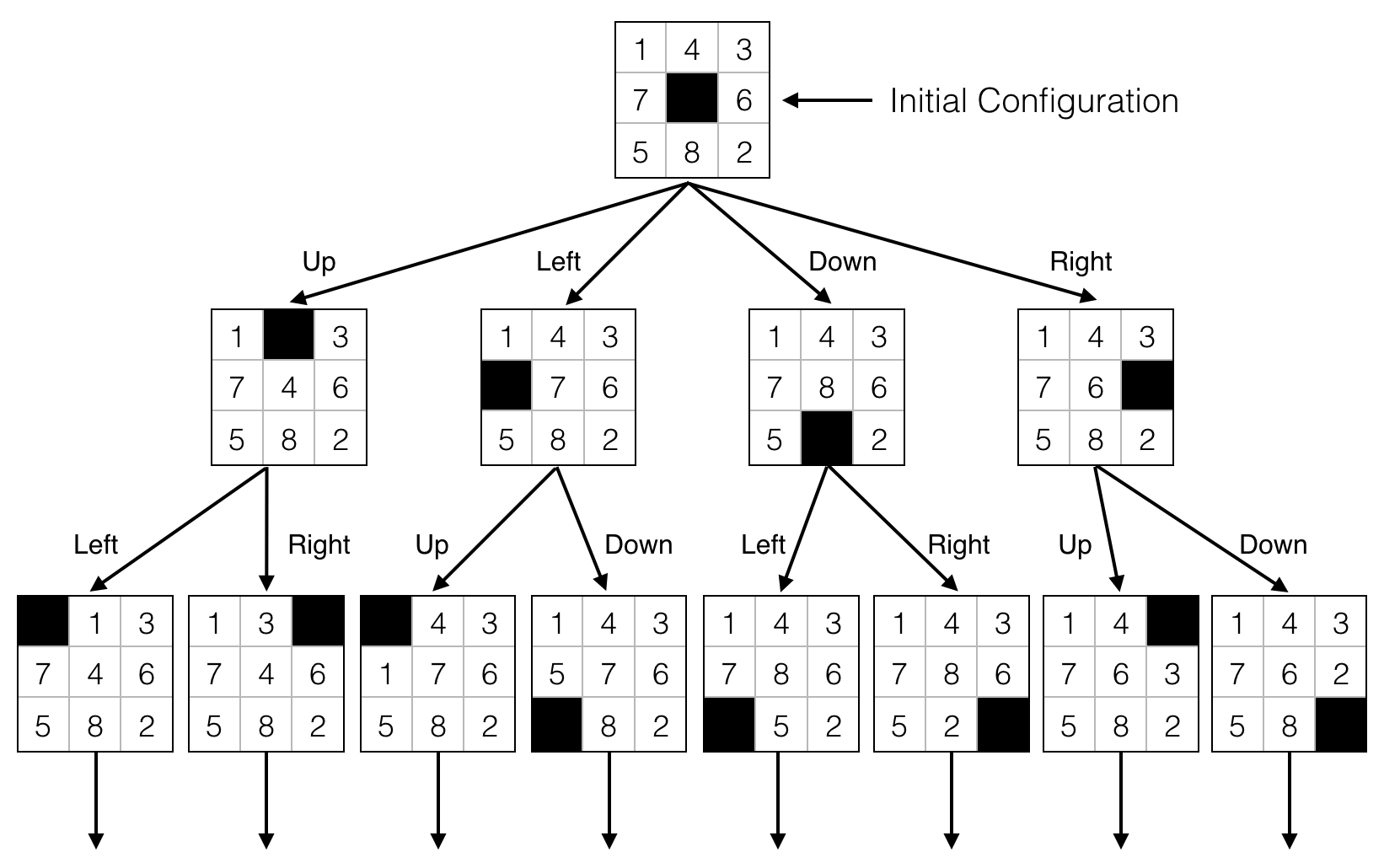This article discusses about using A* Algorithm to solve 24 Puzzle Problem.

# 24 Puzzle

24 Puzzle Problem: 给定一个初始状态和一个目标状态，从初始状态开始，通过空白格的移动，最后达到目标状态。Fig. 1: Goal State 1 - SPRIALFig. 2: Goal State 2 - NORMALFig. 3: The 8-Puzzle state space for a very simple example

# A* Algorithm

A* 算法的估价函数可表示为： $F = G + H$

• $G$ : 初始节点到当前节点的实际代价
• $H$ : 对当前节点到目标节点的距离的启发式估计

• openlist : 待拓展节点的队列，每次搜索都需要所有待拓展节点中 F 最小的那个节点。
• closedlist : 已拓展节点的队列，每个节点存储有它的前驱节点的信息。

# IDA* Algorithm

Iterative Deepening A*

• 启发式搜索算法
• 与 A* 相比，更高效，空间开销更小
• 设置一个与初始状态的启发式估值相等的阈值 (threshold)
• 实施深度优先搜索，当最新节点的估价超过阈值，则剪枝；假如搜索到解，返回它
• 假如没有找到解，将阈值被超过的最小数值加到阈值上，重新开始搜索

# Experiment

• 目标状态：普通 -> $H$: 当前节点与目标节点的曼哈顿距离
• 目标状态：螺旋 -> $H$: Nilsson’s sequence score 尼尔森序列分数 $t = h + 3 × s$
• 假如当前棋盘中心和目标状态的中心不匹配，$s$ 得1分
• 假如当前方块顺时针方向上的第一个方块不是目标状态中它顺时针方向上第一个方块，那么 $s$ 得2分
• $h$ 是当前节点与目标节点的曼哈顿距离

Gif 1: 24 Puzzle Solution - SPRIAL

Gif 2: 24 Puzzle Solution - NORMAL